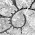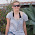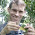## Friday, June 29, 2012

### R trick 1: get the frequencies of factors in a vector

Here's a quick R hint. (I had briefly forgotten how to do this, and the solution wasn't totally obvious online.) Say I have a vector of factors in memory in R and I want to get the frequency or relative frequency of the different levels of the factor, I can do this using the base generic function summary. Just to see how this works, consider a vector containing the best-fitting quantitative trait evolution model for a set of 100 trees:

> best.fit
 BM     BM     OU     lambda BM     BM     OU     BM
 BM     BM     BM     lambda lambda BM     OU     BM
 BM     BM     OU     BM     BM     BM     lambda BM
 BM     BM     BM     OU     lambda BM     BM     BM
 BM     OU     BM     BM     lambda lambda lambda BM
 BM     BM     BM     OU     BM     BM     BM     BM
 BM     OU     BM     BM     BM     BM     BM     BM
 lambda lambda OU     OU     BM     BM     lambda BM
 BM     BM     BM     BM     lambda BM     BM     BM
 BM     OU     OU     BM     lambda BM     lambda BM
 BM     lambda BM     BM     BM     OU     BM     BM
 BM     BM     OU     OU     lambda BM     BM     BM
 BM     BM     OU     BM
Levels: BM lambda OU

We can count up the number or relative frequency of trees with each best fit model as follows:

> summary(best.fit)
BM lambda     OU
68     16     16
> summary(best.fit)/sum(summary(best.fit))
BM lambda     OU
0.68   0.16   0.16

That's it.

1.R trick 2 to follow.

2.Neat! I never use factors much, now I might!

3.but say i have a vector with numbers summary dosn't give me the frequencies it gives me the median, mean, min, max ect.
how do i get the Frequency?

say for simplicity i have this vector: c(1,2,1,4,1,5,6,5,6,5,7)
the frequencies are:  3,  1,  1,  3,  2,  1
But how do i get R to tell me this?

1.This is a hack:

x<-c(1,2,1,4,1,5,6,5,6,5,7)
summary(as.factor(sort(x)))

Alternatively:

obj<-hist(x,breaks=min(x):(max(x)+1)-0.5)
f<-setNames(obj\$counts,obj\$mids)
f

The latter option also gives you any zeroes on the range of your integers.

There may (of course) be some better way to do this that I'm not aware of.

- Liam

4.Note: due to the very large amount of spam, all comments are now automatically submitted for moderation.